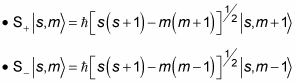##### Quantum Physics For Dummies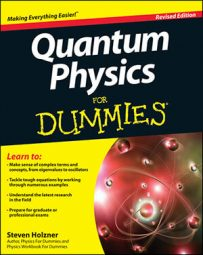Because spin is a type of built-in angular momentum, spin operators have a lot in common with orbital angular momentum operators. As your quantum physics instructor will tell you, there are analogous spin operators, S2 and Sz, to orbital angular momentum operators L2 and Lz. However, these operators are just operators; they don’t have a differential form like the orbital angular momentum operators do.

In fact, all the orbital angular momentum operators, such as Lx, Ly, and Lz, have analogs here: Sx, Sy, and Sz. The commutation relations among Lx, Ly, and Lz are the following: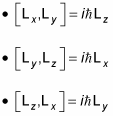And they work the same way for spin: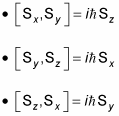The L2 operator gives you the following result when you apply it to an orbital angular momentum eigenstate: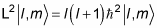And just as you’d expect, the S2 operator works in an analogous fashion: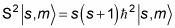The Lz operator gives you this result when you apply it to an orbital angular momentum eigenstate: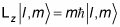And by analogy, the Sz operator works this way: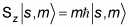What about the raising and lowering operators, L+ and L? Are there analogs for spin? In angular momentum terms, L+ and L work like this: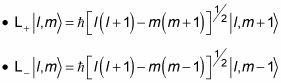There are spin raising and lowering operators as well, S+ and S, and they work like this: